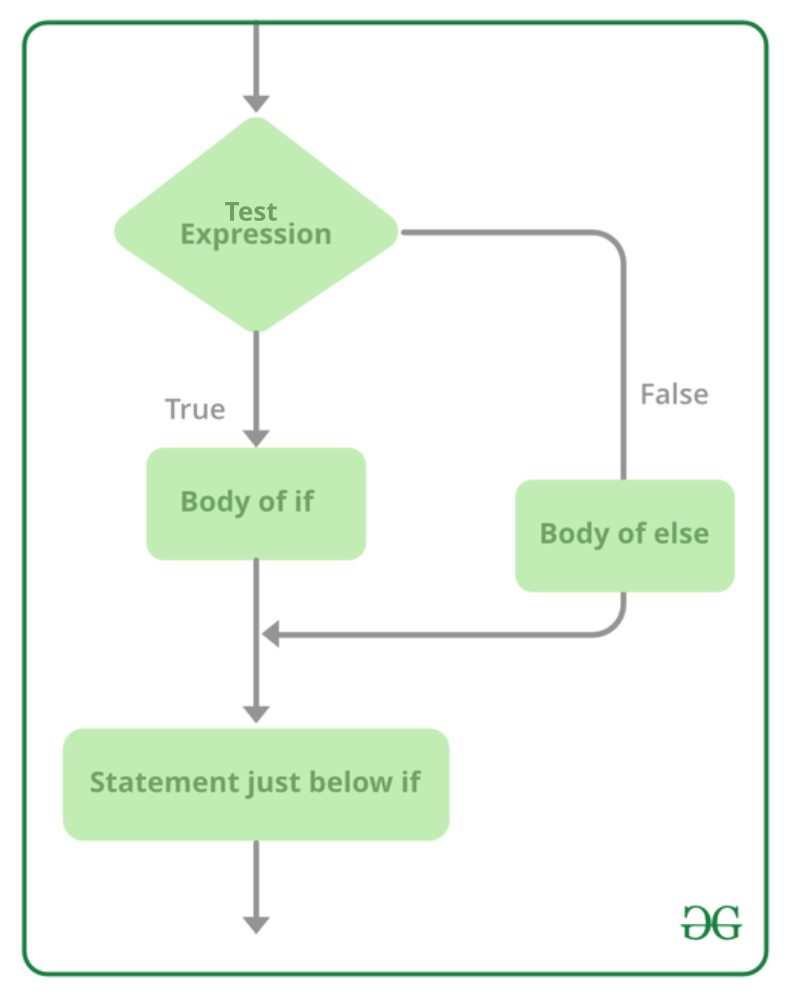# C# – if else Statement

In C#, as we know that if-statement is executed if the condition is true otherwise it will not execute. But, what if we want to print/execute something if the condition is false. Here comes the else statement. Else statement is used with if statement to execute some block of code if the given condition is false. Or in other words, in the if-else statement, if the given condition evaluates to true, then the if condition executes, or if the given condition evaluates to false, then the else condition will execute.

• Else-statement can contain single or multiple statements in the curly braces{}. If the else statement only contains a single statement, then the curly braces are optional.
• The statements of else-statement can be of any kind/type like it may contain another if-else statement.

Syntax:

```if(condition)
{
// code if condition is true
}
else
{
// code if condition is false
}
```

Flow chart:Example 1:

## C#

 `// C# program to demonstrate ` `// if-else statement ` `using` `System; ` ` `  `class` `GFG{ ` `     `  `static` `public` `void` `Main() ` `{ ` `     `  `    ``// Declaring and initializing variables ` `    ``string` `x = ``"Geeks"``; ` `    ``string` `y = ``"GeeksforGeeks"``; ` `     `  `    ``// If-else statement ` `    ``if` `(x == y) ` `    ``{ ` `        ``Console.WriteLine(``"Both strings are equal..!!"``); ` `    ``} ` `     `  `    ``// else statement ` `   ``else` `    ``{ ` `         ``Console.WriteLine(``"Both strings are not equal..!!"``); ` `    ``} ` `} ` `}`

Output:

```Both strings are not equal..!!
```

Example 2:

## C#

 `// C# program to demonstrate if-else statement ` `using` `System; ` ` `  `class` `GFG{ ` `     `  `static` `public` `void` `Main() ` `{ ` `     `  `    ``// Declaring and initializing variables ` `    ``int` `x = 10; ` `    ``int` `y = 100; ` `     `  `    ``// If-else statement ` `    ``if` `(x >= y) ` `    ``{ ` `        ``Console.WriteLine(``"x is greater than y"``); ` `    ``} ` `     `  `    ``// else statement ` `   ``else` `    ``{ ` `         ``Console.WriteLine(``"x is not greater than y"``); ` `    ``} ` `} ` `}`

Output:

```x is not greater than y
```

### Short-Hand of if-else Statement

C# also provides a short-hand implementation of the if-else statement which is also known as Ternary Operator(?:) because it contains three operands. It is basically used to replace multiples lines of codes with a single line. And it will return one of two values depending on the value of a Boolean expression.

Syntax:

```variable_name = (condition) ? TrueExpression :  FalseExpression;
```

Here, if the given condition is true, then the TrueExpression statement will execute. Or if the given condition is false, then the FalseExpression statement will execute.

Note: C# also supports nested Ternary Operator.

Example 1:

## C#

 `// C# program to demonstrate short-hand  ` `// of if-else statement ` `using` `System; ` ` `  `class` `GFG{ ` `     `  `static` `public` `void` `Main() ` `{ ` `     `  `    ``// Declaring and initializing variables ` `    ``string` `x = ``"Geeks"``; ` `    ``string` `y = ``"GeeksforGeeks"``; ` `     `  `    ``// Short-hand if-else statement ` `    ``string` `result = (x == y) ? ``"Both strings are equal"` `: ``"Not equal"``; ` `     `  `    ``// Display result ` `    ``Console.WriteLine(result); ` `} ` `}`

Output:

```Not equal
```

Example 2:

## C#

 `// C# program to demonstrate nested  ` `// Ternary Operator ` `using` `System;  ` ` `  `class` `GFG{  ` `     `  `static` `void` `Main(``string``[] args)  ` `{  ` `     `  `  ``int` `a = 23, b = 90; ` `   `  `  ``string` `result = a > b ? ``"a is greater than b"` `:  ` `                  ``a < b ? ``"a is less than b"` `: ` `                  ``a == b ? ``"a is equal to b"` `: ``"Invalid"``; ` `         `  `  ``Console.WriteLine(result); ` `}  ` `}`

Output:

```a is less than b
```

Whether you're preparing for your first job interview or aiming to upskill in this ever-evolving tech landscape, GeeksforGeeks Courses are your key to success. We provide top-quality content at affordable prices, all geared towards accelerating your growth in a time-bound manner. Join the millions we've already empowered, and we're here to do the same for you. Don't miss out - check it out now!

Previous
Next